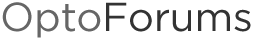# Wireless diagnostic informaiton

Using any of our wireless devices?
Wana get the low-down on the wireless data from the device so you can display it in Pac Display or some other MMI?

If so, then this little bit of OptoScript is for you;

``````

//Read the Signal to Noise ratio from the wireless NIC
iWireless_Trash = ReadNumFromIoUnitMemMap(IO_Unit, 0xF7003040, iWireless_StoN_Ratio_IO_Unit);

iWireless_Trash = ReadNumFromIoUnitMemMap(IO_Unit, 0xF7003034, iWireless_Frequency_IO_Unit);

iWireless_Trash = ReadNumFromIoUnitMemMap(IO_Unit, 0xF7003038, iWireless_Rx_Rate_IO_Unit);

//Read the Transmit Rate
iWireless_Trash = ReadNumFromIoUnitMemMap(IO_Unit, 0xF700303C, iWireless_Tx_Rate_IO_Unit);

iWireless_Trash = ReadNumFromIoUnitMemMap(IO_Unit, 0xF700305C, iWireless_Pkts_Rx_IO_Unit);

//Read the Packets Transmitted
iWireless_Trash = ReadNumFromIoUnitMemMap(IO_Unit, 0xF7003068, iWireless_Pkts_Tx_IO_Unit);

//Read the Packets Transmit Problems
iWireless_Trash = ReadNumFromIoUnitMemMap(IO_Unit, 0xF7003070, iWireless_Pkts_Tx_Problems_IO_Unit);

//Calculate Bad Receive Error Rate in Percent
fWireless_Temp_Error_Rate_1 = iWireless_Pkts_Rx_Bad_IO_Unit * 1.0; //Convert INTs to Floats
fWireless_Temp_Error_Rate_2 = iWireless_Pkts_Rx_IO_Unit * 1.0;     //Convert INTs to Floats
fWireless_Temp_Error_Rate_3 = fWireless_Temp_Error_Rate_1 / fWireless_Temp_Error_Rate_2;  //Divide to determine error rate
fWireless_Pkts_Rx_Error_Rate_IO_Unit = fWireless_Temp_Error_Rate_3 * 100;                //Multiply by 100 to get percent

//Calculate Bad Transmit Error Rate in Percent
fWireless_Temp_Error_Rate_1 = iWireless_Pkts_Tx_Problems_IO_Unit * 1.0; //Convert INTs to Floats
fWireless_Temp_Error_Rate_2 = iWireless_Pkts_Tx_IO_Unit * 1.0;     //Convert INTs to Floats
fWireless_Temp_Error_Rate_3 = fWireless_Temp_Error_Rate_1 / fWireless_Temp_Error_Rate_2;  //Divide to determine error rate
fWireless_Pkts_Tx_Error_Rate_IO_Unit = fWireless_Temp_Error_Rate_3 * 100;                //Multiply by 100 to get percent

iWireless_Trash = ReadStrFromIoUnitMemMap(20, IO_Unit, 0xF7003014, sWireless_SSID_IO_Unit);

//Read status of wireless connection;
//(WLAN state: 0 = disconnected, 1 = inactive, 2 = scanning, 3 = associating, 4 = associated, 5 = wpa 4-way handshake, 6 = wpa group handshake, 7 = completed):

iWireless_Trash = ReadNumFromIoUnitMemMap(IO_Unit, 0xF7003008, iWireless_ConnStatus_IO_Unit);

Switch (iWireless_ConnStatus_IO_Unit)
Case 0:
sWireless_ConnStatus_IO_Unit = "Disconnected";
Break
Case 1:
sWireless_ConnStatus_IO_Unit = "Inactive";
Break
Case 2:
sWireless_ConnStatus_IO_Unit = "Scanning";
Break
Case 3:
sWireless_ConnStatus_IO_Unit = "Associating";
Break
Case 4:
sWireless_ConnStatus_IO_Unit = "Associated";
Break
Case 5:
sWireless_ConnStatus_IO_Unit = "WPA 4-way Handshake";
Break
Case 6:
sWireless_ConnStatus_IO_Unit = "WPA Group Handshake";
Break
Case 7:
sWireless_ConnStatus_IO_Unit = "Completed";
Break
Endswitch

//Read the MAC address of the WAP, build it as a string.

NumberToHexString((iWireless_MAC1 bitand 0xFF000000) >> 24, sWireless_MAC_Temp);
if (GetStringLength(sWireless_MAC_Temp) == 1) then sWireless_MAC_Temp = "0" + sWireless_MAC_Temp ; endif
sWireless_WAP_MAC_Address = sWireless_WAP_MAC_Address + sWireless_MAC_Temp + "-";

NumberToHexString((iWireless_MAC1 bitand 0x00FF0000) >> 16, sWireless_MAC_Temp);
if (GetStringLength(sWireless_MAC_Temp) == 1) then sWireless_MAC_Temp = "0" + sWireless_MAC_Temp ; endif
sWireless_WAP_MAC_Address = sWireless_WAP_MAC_Address + sWireless_MAC_Temp + "-";

NumberToHexString((iWireless_MAC1 bitand 0x0000FF00) >>  8, sWireless_MAC_Temp);
if (GetStringLength(sWireless_MAC_Temp) == 1) then sWireless_MAC_Temp = "0" + sWireless_MAC_Temp ; endif
sWireless_WAP_MAC_Address = sWireless_WAP_MAC_Address + sWireless_MAC_Temp + "-";

NumberToHexString((iWireless_MAC1 bitand 0x000000FF)      , sWireless_MAC_Temp);
if (GetStringLength(sWireless_MAC_Temp) == 1) then sWireless_MAC_Temp = "0" + sWireless_MAC_Temp ; endif
sWireless_WAP_MAC_Address = sWireless_WAP_MAC_Address + sWireless_MAC_Temp + "-";

NumberToHexString((iWireless_MAC2 bitand 0xFF000000) >> 24, sWireless_MAC_Temp);
if (GetStringLength(sWireless_MAC_Temp) == 1) then sWireless_MAC_Temp = "0" + sWireless_MAC_Temp ; endif
sWireless_WAP_MAC_Address = sWireless_WAP_MAC_Address + sWireless_MAC_Temp + "-";

NumberToHexString((iWireless_MAC2 bitand 0x00FF0000) >> 16, sWireless_MAC_Temp);
if (GetStringLength(sWireless_MAC_Temp) == 1) then sWireless_MAC_Temp = "0" + sWireless_MAC_Temp ; endif

``````

Cut and paste that into an OptoScipt block, run it every second or so (just often enough to get a regular update).
You probably will want to do a find and replace and change all the ‘IO_Unit’ tags for the name of your I/O unit (or controller).
If there is more information you need, then just tack it on the bottom, this should be more than enough to get you started.

Have you got any code that gets any sort of diagnostic information out of a controller or brain that you would like to share?
Post it here, or if you like, you can email me and we can build up a post together.

In the mean time,

Code On!